## Introduction to Power Series

It often happens that a differential equation cannot be solved in terms of elementary functions (that is, in closed form in terms of polynomials, rational functions, e x , sin x, cos x, In x, etc.). A power series solution is all that is available. Such an expression is nevertheless an entirely valid solution, and in fact, many specific power series that arise from solving particular differential equations have been extensively studied and hold prominent places in mathematics and physics.

A power series in x about the point x 0 is an expression of the form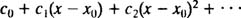where the coefficients c n are constants. This is concisely written using summation notation as follows:Attention will be restricted to x 0 = 0; such series are simply called power series in x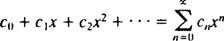A series is useful only if it converges (that is, if it approaches a finite limiting sum), so the natural question is, for what values of x will a given power series converge? Every power series in x falls into one of three categories:

•

The power series converges only for x = 0.

• Category 2:

The power series converges for | x| < R and diverges (that is, fails to converge) for | x| > R (where R is some positive number).

• Category 3:

The power series converges for all x.

Since power series that converge only for x = 0 are essentially useless, only those power series that fall into Category 2 or Category 3 will be discussed here.

The ratio test says that the power series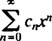will converge if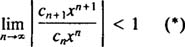and diverge if this limit is greater than 1. But (*) is equivalent toso the positive number R mentioned in the definition of a Category 2 power series is this limit: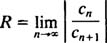If this limit is ∞, than the power series converges for | x| < ∞—which means for all x—and the power series belongs to Category 3. R is called the radius of convergence of the power series, and the set of all x for which a real power series converges is always an interval, called its interval of convergence.

Example 1: Find the radius and interval of convergence for each of these power series: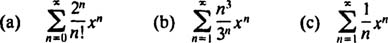[Recall that n! (“ n factorial”) denotes the product of the positive integers from 1 to n. For example, 4! = 1 · 2 · 3 · 4 = 25 By definition, 0! is set equal to 1.]

a. In this power series, c n = 2 n / n!, so the ratio test saysTherefore, this series converges for all x.

b. The radius of convergence of the power series in (b) is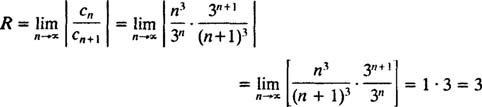Since R = 3, the power series converges for | x| < 3 and diverges for | x| > 3. For a power series with a finite interval of convergence, the question of convergence at the endpoints of the interval must be examined separately. It may happen that the power series converges at neither endpoint, at only one, or at both. The power series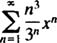converges at neither the endpoint x = 3 nor x = −3 because the individual terms of both resulting series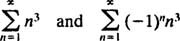clearly do not approach 0 as n → ∞. (For any series to converge, it is necessary that the individual terms go to 0.) Therefore, the interval of convergence of the power series in (b) is the open interval −3 < x < 3.

c. The radius of convergence of this power series isSince R = 1, the seriesconverges for | x| < 1 and diverges for | x| > 1. Since this power series has a finite interval of convergence, the quetion of convergence at the endpoints of the interval must be examined separately. At the endpoint x = −1, the power series becomes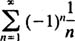which converges, since it is an alternating series whose terms go to 0. However, at the endpoint x = 1, the power series becomeswhich is known to diverge (it is the harmonic series). Therefore, the interval of convergence of the power series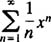is the half‐open interval −1 ≤ x < 1.

Back to Top
A18ACD436D5A3997E3DA2573E3FD792A